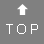# How to calculate your partner rune

Write your name on a piece of paper. Match every character with the right number, which you find below. Generate the cross sum. If the sum is higher than 24, repeat the procedure until you will get a value between 1 and 24.

 A B C D E F G H I J K L M N 4 18 6 23 19 1 7 9 11 12 6 21 20 10 O P Q R S T U V W X Y Z NG TH 24 14 8 5 16 17 2 8 8 22 12 15 22 3
Do the same with the name of your partner. Than add the sum totals until you get a value between 1 and 24.

For example: Your name is Helen Jordan.

 H e l e n J o r d a n 9 19 21 19 10 12 24 5 23 4 10

first sum = 9 + 19 + 21 + 19 + 10 + 12 + 24 + 5 + 23 + 4 + 10 = 156
checksum = 1 + 5 + 6 = 12

For example: The name of your partner is Marc Cain

 M a r c C a i n 20 4 5 6 6 4 11 10

first sum = 20 + 4 + 5 + 6 + 6 + 4 + 11 + 10 = 66
checksum = 6 + 6 = 12

12 + 12 = 24 - The partner rune is Othala (24)

Look here to see the results. ... or use the online-calculator for the partner rune.

How - 2022-11-08 21:36:05
How do you sum it should be less complex

Total 1 posts